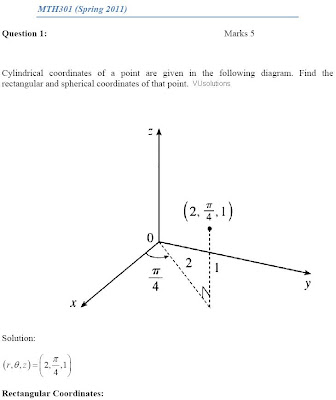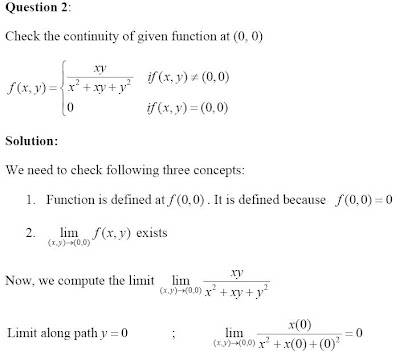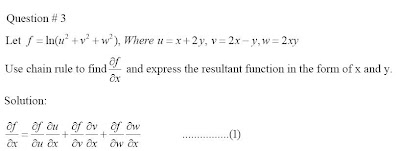# Thread: mth301 assignment no 1 spring 2011 solution on vuhelp.net

1. ##mth301 assignment no 1 spring 2011 solution on vuhelp.net

Assignment # 1
MTH301 (Spring 2011)
Question 1: Marks 5
Cylindrical coordinates of a point are given in the following diagram. Find the
rectangular and spherical coordinates of that point.

Solution:
 , ,  2, ,1
4
r z

   
 
Rectangular Coordinates:
   
cos 2cos 2 1 2
4 2
sin 2sin 2 1 2
4 2
1
, , 2, 2,1
x r
y r
z z
x y z

       
 
       
 
 
 
Spherical Coordinates:
 
 
2 2 2 2
1 1
, , 2, ,1
4
(2) (1) 5
4
tan tan 2 1.107
1
, , 5, ,1.107
4
r z
r z
r
z

 

  
 
    
 
    
 
          
   
     
 
Question 2: Marks 05
Check the continuity of given function at (0, 0)
2 2 ( , ) (0,0)
( , )
0 (, ) (0,0)
xy if x y
f x y x xy y
if x y
      
  
Solution:
We need to check following three concepts:
1. Function is defined at f (0,0) . It is defined because f (0,0)  0
2.
( , ) (0,0)
lim ( , )
x y
f x y

exists
Now, we compute the limit ( , ) (0,0) 2 2
lim
x y
xy
 x  xy  y
( , ) (0,0) 2 2
Limit along path 0 ; lim (0) 0
x y (0) (0)
y x
 x x
 
 
( , ) (0,0) 2 2
Limit along path 0 ; lim (0) 0
x y (0) (0)
x y
 y y
 
 
2 2
( , ) (0,0) 2 2 2 2 2 2
Limit along path
lim ( ) 1
x y ( ) ( ) 3 3
y x
x x x x
 x x x x x x x x

  
   
Since, we are getting different values along different paths, therefore,
( , ) (0,0) 2 2
lim
x y
xy
 x  xy  y
does not exist.
There is a third condition too to check continuity, and it is that
( , ) (0,0)
(0,0) lim ( , )
x y
f f x y

 ,
but since
( , ) (0,0)
lim ( , )
x y
f x y

does not exist, therefore, there is no need to check third
condition.
Conclusion:
Given function is discontinuous at (0,0)
Question # 3 Marks 05
Let f  ln(u2  v2  w2 ), Where u  x  2y, v  2x  y,w  2xy
Use chain rule to find f
x

and express the resultant function in the form of x and y.
Solution:
f f u f v f w ................(1)
x u x v x w x
      
  
      
 
 
 
2 2 2
2 2 2
2 2 2
2 2 2
2 2 2
2 2 2
ln( ) 2
2 1
ln( ) 2
2 2
ln( ) 2
2 2
f u v w u
u u u v w
u x y
x x
f u v w v
v v u v w
v x y
x x
f u v w w
w w u v w
w xy y
x x
             
 
  
 
             
 
  
 
             
 
 
 
Put all derivatives in Eq. (1), we get
 
2 2 2 2 2 2 2 2 2
2 2 2 2 2 2 2 2 2
2 2 2
2 (1) 2 (2) 2 (2 )
2 4 4
1 2 4 4
f u v wy
x u v w u v w u v w
f u v wy
x u v w u v w u v w
f u v wy
x u v w

  
      

  
      

  
  Reply With Quote

2.Reply With Quote

3. ##find the attachment for the solutionReply With Quote